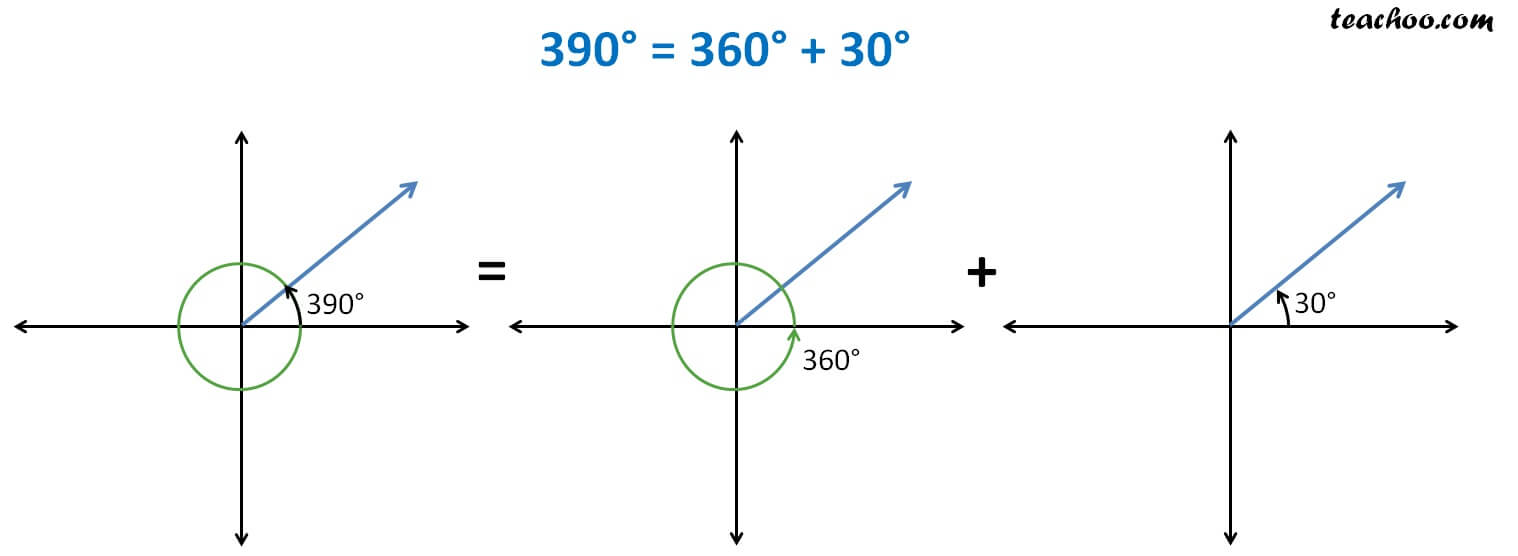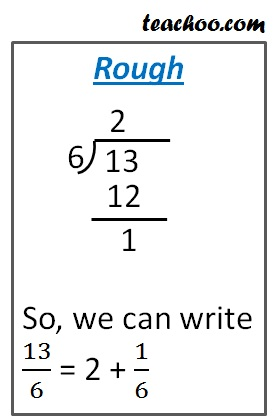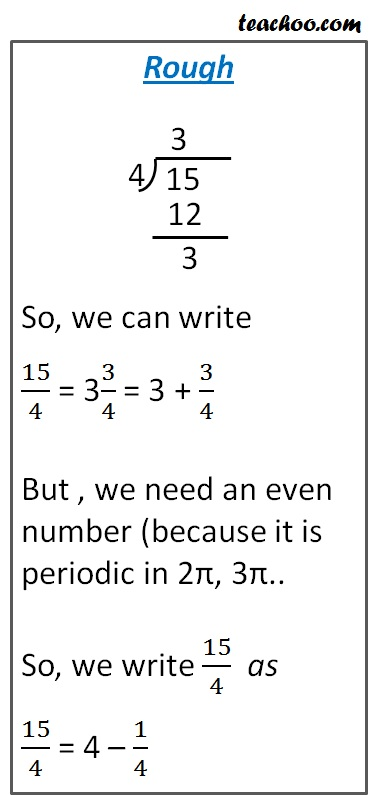Finding Value of trignometric functions, given angle

Chapter 3 Class 11 Trigonometric Functions
Concept wise

sin (2π + x) = sin x

cos (2π + x) = cos x

tan (2π + x) = tan x

Here x is an acute angle.

and 2π = 2 × 180° = 360°

Let’s see why there are same.

Suppose x = 390°

x = 360° + 30°We note that

x = 390° is the same as 30°

So, we can write

cos (390°) =  cos (30°)

sin (390°) =  sin (30°)

tan (390°) =  tan (30°)

Similarly,

if the angle was

x = 750°

x = 2 × 360° + 30°

Thus, we rotate twice and come back to 30°

∴ x = 750° is the same as 30°

So, we can write

cos (750°) =  cos (30°)

sin (750°) =  sin (30°)

tan (750°) =  tan (30°)

Thus, we observe that

sin (n × 360° + x) = sin x

where n = 1, 2, 3, 4, ….

sin (n × 2π + x) = sin x

cos (n × 2π + x) = cos x

tan (n × 2π + x) = tan x

where n = 1, 2, 3, 4, ….

Note: For sec, cosec, cot … we convert them into sin, cos, tan and apply the sin, cos, tan formula

Let’s try some questions

### Find cos 390°

First, we convert 390° into radians.

We multiply by π/180

x = 390  × π/180

x = π × 390/180

x = π × 13/6

x = 13π/6

So, cos 390° = cos 13π/6Thus,

cos 13π/6

=  cos (2π + π/6)

Since values of cos x repeats after an interval of 2π ,hence ignoring

= cos (π/6)

= cos (180/6°)

= cos 30°

= √3/2

### Find value of tan (–15 π /4)

tan (–15π/4)

As tan (–x) = – tan x

= – tan (15π/4)= – tan (4π – π/4)

= – tan (–π/4)

As tan (–x) = – tan x

= – (– tan (π/4))

= tan (π/4)

= tan (180/4 °)

= tan (45°)

= 1

∴ tan (–15π/4) = 1

Get live Maths 1-on-1 Classs - Class 6 to 12

### Transcript

sin (2 + x) = sin x cos (2 + x) = cos x tan (2 + x) = tan x Here x is an acute angle. and 2 = 2 180 = 360 Let s see why there are same. Suppose x = 390 x = 360 + 30 We note that x = 390 is the same as 30 So, we can write cos (390 ) = cos (30 ) sin (390 ) = sin (30 ) tan (390 ) = tan (30 ) Similarly, if the angle was x = 750 x = 2 360 + 30 Thus, we rotate twice and come back to 30 x = 750 is the same as 30 So, we can write cos (750 ) = cos (30 ) sin (750 ) = sin (30 ) tan (750 ) = tan (30 ) Thus, we observe that sin (n 360 + x) = sin x where n = 1, 2, 3, 4, . Writing in radians sin (n 2 + x) = sin x cos (n 2 + x) = cos x tan (n 2 + x) = tan x where n = 1, 2, 3, 4, . Note: For sec, cosec, cot we convert them into sin, cos, tan and apply the sin, cos, tan formula Let s try some questions Find cos 390 First, we convert 390 into radians. We multiply by /180 x = 390 /180 x = 390/180 x = 13/6 x = 13 /6 So, cos 390 = cos 13 /6 Thus, cos 13 /6 = cos (2 + /6) Since values of cos x repeats after an interval of 2 ,hence ignoring 2 = cos ( /6) = cos (180/6 ) = cos 30 = 3/2 Find value of tan ( 15 /4) tan ( 15 /4) As tan ( x) = tan x = tan (15 /4) = tan (4 /4) = tan ( /4) As tan ( x) = tan x = ( tan ( /4)) = tan ( /4) = tan (180/4 ) = tan (45 ) = 1 tan ( 15 /4) = 1